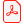# Konferensartikel

## Methods for Some Linear and Quadratic Optimization Problems Defined on a Set of Orthogonal Vectors

Per-åke Wedin
Umeå University, Sweden

Ladda ner artikelLinköping Electronic Conference Proceedings 14:31, s.

Visa mer +

ISBN:

ISSN: 1650-3686 (tryckt), 1650-3740 (online)

### Abstract

For several practical optimization problems one wants to find a minmizer that belongs to a set of orthonormal vectors. In most cases these problems are 3-dimensional and related to rigid body movement; tereophotogrammetry and similar applications. However;there are also; e.g. in psychometrics; quadratic or linear optimization problems over a set of m*n-matrices Q with orthonormal columns a.k.a. a Stiefel manifold. Interesting properties that set problems of this kind apart are the following: (1) The function to be minimized is nice. It is always convex; while the set that we optimize over is non-convex and fairly tricky. (2) It is possible to get a useful unconstrained LOCAL representation of the optimization problem while the constrained representation is needed globally. (3) Some optimization problems of this kind; e.g. the Procrustes problem; have a unique minimum thar can be attained by using the singular value decomposition. (4) There are several optimization problems of this kind where the number of local minima will grow very fast with the number of orthogonal vectors to be optimized over. (5) Geometrical considerations combined with Lagrange multipier theory are useful analytic tools.

Thomas Viklands; Umeå; has developed an algorithm and furthered the analysis for one problem of this kind; the Penrose-Procrustes regression problem. Viklands has shown that this problem can have 2n local minima. Here n is the number of orthogonal vectors for which the optimization problem is defined. Vikland´s algorithm tries to find all the minima of the P-P problem.

In this talk we will summarize the state of the art of the research in this area with special emphasis on the Penrose-Procrustes regression problem.

### Nyckelord

Inga nyckelord är tillgängliga

### Referenser

Inga referenser tillgängliga